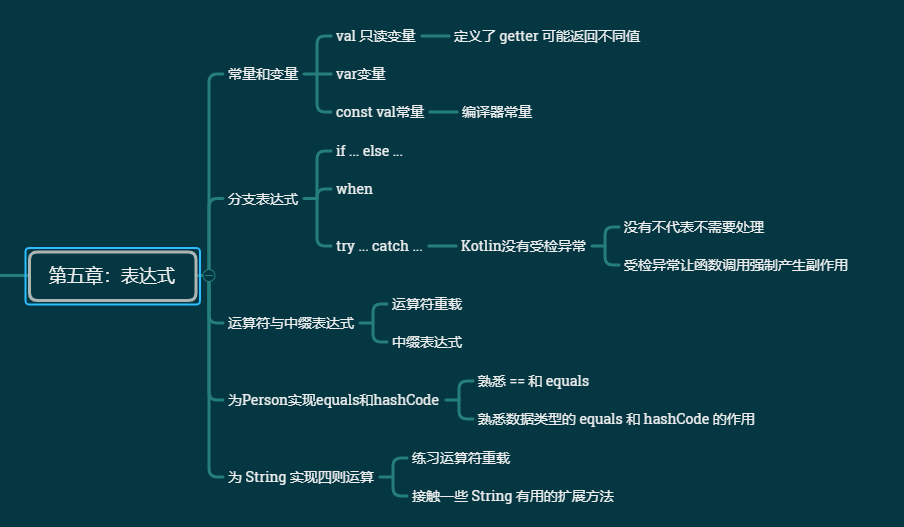Java

Kotlin

Java

Kotlin

Java

Kotlin

• 只能定义在全局范围
• 只能修饰基本类型
• 必须立即用字面量初始化

# 表达式

## if … else

Java

Java支持三目运算符，所以可以使用以下语法

Kotlin

Kotlin中，`if ... else ...`也属于表达式，所以Kotlin开发者没有提供三目运算符，直接使用`if ... else ...`可以达到相同的效果

Java

Kotlin

Java

Kotlin

# 运算符与中缀表达式

## 运算符

• Kotlin支持运算符重载
• 运算符的范围仅限官方指定的符号

### 一元操作

#### 一元前缀操作符

表达式 翻译为
+a a.unaryPlus()
-a a.unaryMinus()
!a a.not()

1. 确定`a`的类型，令其为`T`
2. 为接收者`T`查找一个带有`operator`修饰符的无参函数`unaryPlus()`，即成员函数或扩展函数；
3. 如果函数不存在或不明确，则导致编译错误；
4. 如果函数存在且其返回类型为`R`，那就表达式`+a`具有类型`R`

a++ a.inc()
a– a.dec()

### 二元操作

#### 算术运算符

a + b a.plus(b)
a - b a.minus(b)
a * b a.times(b)
a / b a.div(b)
a % b a.rem(b) 或者 a.mod(b) 已弃用
a..b a.rangeTo(b)

#### `In`操作符

a in b b.contains(a)
a !in b !b.contains(a)

#### 索引访问操作符

a[i] a.get(i)
a[i, j] a.get(i, j)
a[i_1, ……, i_n] a.get(i_1, ……, i_n)
a[i] = b a.set(i, b)
a[i, j] = b a.set(i, j, b)
a[i_1, ……, i_n] = b a.set(i_1, ……, i_n, b)

#### 调用操作符

a() a.invoke()
a(i) a.invoke(i)
a(i, j) a.invoke(i, j)
a(i_1, ……, i_n) a.invoke(i_1, ……, i_n)

#### 广义赋值

a += b a.plusAssign(b)
a -= b a.minusAssign(b)
a *= b a.timesAssign(b)
a /= b a.divAssign(b)
a %= b a.remAssign(b), a.modAssign(b)（已弃用）

#### 相等与不等操作符

a == b a?.equals(b) ?: (b === null)
a != b !(a?.equals(b) ?: (b === null))

#### 比较操作符

a > b a.compareTo(b) > 0
a < b a.compareTo(b) < 0
a >= b a.compareTo(b) >= 0
a <= b a.compareTo(b) <= 0

Java(since 1.8)

Kotlin

Java(since 1.8)

Kotlin